\$B:#>,\$N0lGU(B

\$BKhF|!":#>,\$N0lGU\$r>e\$2\$F\$\$\$^\$9!#(B
\$B:#>,0{\$`\$b\$N\$K:\$\$C\$?\$i!";29M\$K\$7\$F\$_\$F\$/\$@\$5\$\$!#(B

2020\$BG/(B11\$B7n(B

 1 \$B5Y\$_(B 2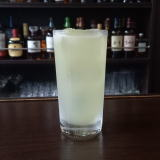(I 3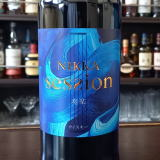(IF/6\$B!!(I>/<.](B 4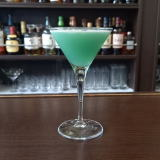(I=62E2D%K^-0(B 5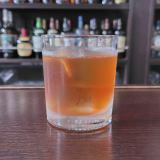(I[0O%NXC^0(B 6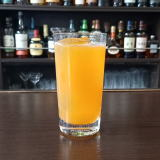(IN^;I3^'(B 7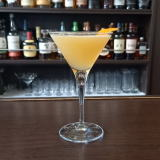(IA,M_Y%KY(B 8 \$B5Y\$_(B 9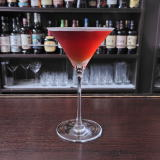(I<^,]8^Y%68CY(B 10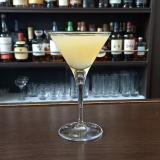(IW=D%7/=(B 11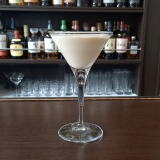(I\]%S1%L+0%;^%[0D^(B 12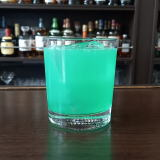(I8^X0]%C^K^Y(B 13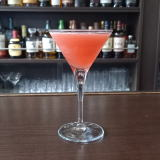(I<^]%=7](B 14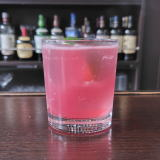(I\2YD^%<]8^(B 15 \$B5Y\$_(B 16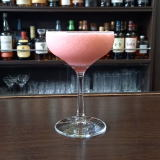(IOAY@^(B 17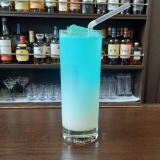\$B%O%o%\$%"%s!&%J%\$%H(B 18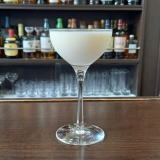(IQ0]%8^[0(B 19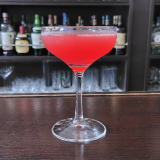(IK_]8%J_Z=(B 20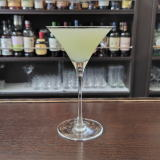(IC28%L'2L^(B 21(I6XS0A.(B 22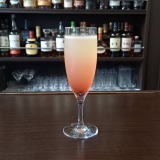(I=0<^0%3+](B 23 \$B5Y\$_(B 24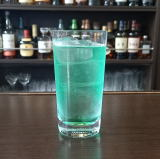(I8^X0]%J/D(B 25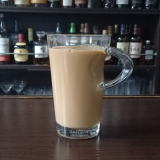(IM^2X0=^%:0K0(B 26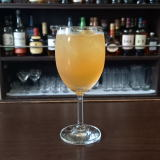(I<,]J_]%80W0(B 27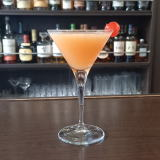(IW2QW2D(B 28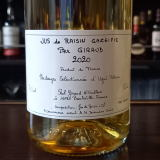(IN_0Y%<^[0\$B!!(I<^-0=(B 29 \$B5Y\$_(B 30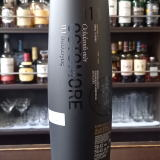(I58DS1(B11.1